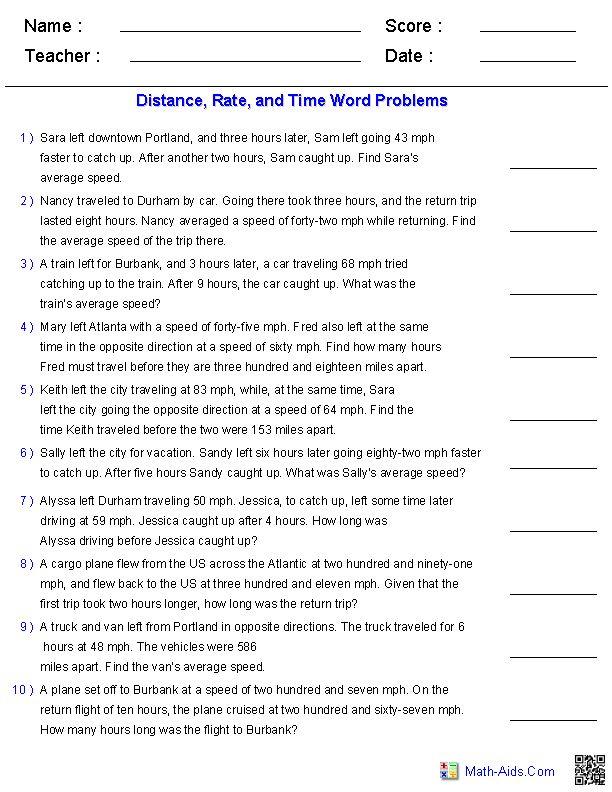Algebra 1 Worksheets

Word Problems Worksheets

Here is a graphic preview for all of the Word Problems Worksheets. You can select different variables to customize these Word Problems Worksheets for your needs. The Word Problems Worksheets are randomly created and will never repeat so you have an endless supply of quality Word Problems Worksheets to use in the classroom or at home. Our Word Problems Worksheets are free to download, easy to use, and very flexible.

These Word Problems Worksheets are a good resource for students in the 5th Grade, 6th Grade, 7th Grade, and 8th Grade.

Click here for a Detailed Description of all the Word Problems Worksheets.

Quick Link for Word Problems Worksheets

Click the image to go to that Word Problems Worksheets.

One Step EquationWord ProblemsTwo Step EquationWord ProblemsDistance, Rate, and TimeWord ProblemsMixture Word ProblemsWork Word ProblemsRecommended Videos

Description of Word Problems Worksheets

One Step Equation Word Problems
These Algebra 1 Equations Worksheets will produce one step word problems. These worksheets will produce ten problems per worksheet. These Equations Worksheets are a good resource for students in the 5th Grade through the 8th Grade.

Two Step Equation Word Problems
These Algebra 1 Equations Worksheets will produce two step word problems. These worksheets will produce ten problems per worksheet. These Equations Worksheets are a good resource for students in the 5th Grade through the 8th Grade.

Distance, Rate, and Time Word Problems
These Algebra 1 Equations Worksheets will produce distance, rate, and time word problems with ten problems per worksheet. You may select the numbers to be represented with digits or in words. These Equations Worksheets are a good resource for students in the 5th Grade through the 8th Grade.

Mixture Word Problems
These Algebra 1 Equations Worksheets will produce mixtures word problems with ten problems per worksheet. You may select the numbers to be represented with digits or in words. These Equations Worksheets are a good resource for students in the 5th Grade through the 8th Grade.

Work Word Problems
These Algebra 1 Equations Worksheets will produce work word problems with ten problems per worksheet. You may select the numbers to be represented with digits or in words. These Equations Worksheets are a good resource for students in the 5th Grade through the 8th Grade.